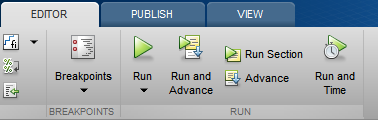## Loren on the Art of MATLABTurn ideas into MATLAB

Note

Loren on the Art of MATLAB has been archived and will not be updated.

Well, it's about that time. What time? It turns out that this is my 400th post. In 10 years! It's been a lot of fun for me; and I hope you've learnd a lot. So time to talk about time again.

### Contents

#### Timing before computers

There are a bunch of ways to time code execution in MATLAB. Of course, you could always use a stopwatch when you start to run some code, and, assuming it takes long enough, but not so long that you lose interest, you might get reasonable estimate. However, many computations happen very fast on a human timescale, and so we want a way to measure the time more accurately.

#### Timing 1.0

When I started using MATLAB, we performed timings using tic and toc. Like this:

a = rand(1000);
tic, b = a*a*a*a; elapsedtic = toc

elapsedtic =
0.17972


The good thing about tic and toc is that they help you measure the time you wait before the prompt reappears. So if your computer tries to go do something else while the computation is going on, you may incorrectly see a much larger time than the time actually spent on your calculation. To mitigate that, we introduced cputime. In the days of single core machines, the original tic-toc times and cputime should have been about the same. Unless, of course, your computer was multitasking majorly in the background. Then cputime might be more reflective of the actual computation time required. But even then, we thought tic and toc were more what people wanted to know, and recommended it in the documentation.

t = cputime; c = a*a*a*a; elapsedcpu = cputime-t

elapsedcpu =
0.4212


But wait! elapsedcpu is much larger than elapsedtic, by a factor of

ratio = elapsedcpu/elapsedtic


What's going on? Well, I happen to be running this example on a dual core machine... So, for functionality that is multithreaded, meaning much of the math in MATLAB, the cputime calculation is likely to appear larger by a factor of the number of cores you have access to.

ratio =
2.3437


#### Timing accuracy

Even with all of these timing tools, and with next advance being the introduction of profiling in MATLAB (which you can reach from the EDITOR Toolstrip), we still saw a lot of variability in timing.Some of this variability had to do with details of how the MATLAB execution engine works. And whether code could be sped up by the JIT or Accelerator (old technology now compared to R2015b!). Some of the variablity could be alleviated by running the code in functions that MATLAB can interpret and "compile" first, and then re-use to run again without having to re-interpret the code. To smooth out the costs of the first time overhead, we introduced timeit.

timeittime = timeit(@() a*a*a*a)


So now you can understand the doc excerpt below. While true, it's nice to know why!

  Although it is possible to measure performance using the cputime
function, it is recommended that you use the timeit or tic and toc
functions for this purpose exclusively.
timeittime =
0.14095


#### GPU Timing

If you happen to have access to GPUs for your computations, you may want to check out gputimeit.

#### Ten Years

So that's where the time has gone. Hope yours has been productive! And have an enjoyable holiday season and happy new year!

Published with MATLAB® R2015b

### コメント

コメントを残すには、ここ をクリックして MathWorks アカウントにサインインするか新しい MathWorks アカウントを作成します。